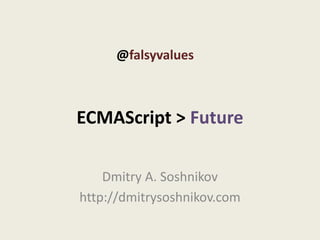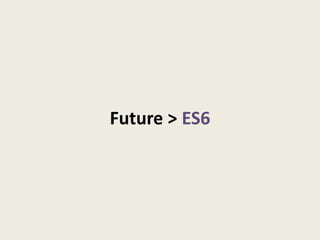Successfully reported this slideshow.

# FalsyValues. Dmitry Soshnikov - ECMAScript 6

40

Share×
1 of 58
1 of 58

# FalsyValues. Dmitry Soshnikov - ECMAScript 6

40

Share

@falsyvalues on 2011-05-20, Warsaw. Dmitry Soshnikov - ECMAScript 6 aka "Harmony".

@falsyvalues on 2011-05-20, Warsaw. Dmitry Soshnikov - ECMAScript 6 aka "Harmony".

## More Related Content

### Related Books

Free with a 14 day trial from Scribd

See all

### Related Audiobooks

Free with a 14 day trial from Scribd

See all

### FalsyValues. Dmitry Soshnikov - ECMAScript 6

1. 1. @falsyvalues ECMAScript > Future Dmitry A. Soshnikov http://dmitrysoshnikov.com
2. 2. Future > ES6
3. 3. let : block scope
4. 4. Block scope // ES3 if (false) { var logLevel = 10; } alert(logLevel); // ?
5. 5. Block scope // ES3 var logLevel; // = undefined; All variables are if (false) { created before code execution – on logLevel = 10; entering the context. } A so-called “hoisting” of variables. alert(logLevel); // undefined See: http://dmitrysoshnikov.com/notes/note-4-two-words-about-hoisting/
6. 6. Block scope // ES6 Harmony if (false) { let logLevel = 10; } alert(logLevel); // ReferenceError
7. 7. Block scope // ES3, ES5 var handlers = []; handlers(); // ? handlers(); // ? for (var k = 0; k < 3; k++) { handlers(); // ? handlers[k] = function () { alert(k); }; }
8. 8. Block scope // ES3, ES5 var handlers = []; handlers(); // 3 var k; handlers(); // 3 for (k = 0; k < 3; k++) { handlers(); // 3 handlers[k] = function () { alert(k); }; }
9. 9. let : block scope ES3, ES5 ES6 for (var k = 0; k < 3; k++) { for (let k = 0; k < 3; k++) { let x = k; (function (x) { handlers[x] = function () { handlers[x] = function () { alert(x); alert(x); }; }; } })(k); } handlers(); // 0 handlers(); // 0
10. 10. let : definition, statement, expression let x = 10; // let-definition let y = 20; let (x = x * 2, y = 30) { console.log(x + y); // 50 // let-statement } console.log(x + y); // 30 console.log(let (x = 100) x); // 100 // let-expression console.log(x); // 10
11. 11. const : constants
12. 12. const : constants const MAX_SIZE = 100; // cannot be redeclared (let, var, etc.) let MAX_SIZE = 100; // error
13. 13. const : constant functions const registerUser() { // implementation } // redeclaration error (function, let, var) function registerUser() { ... }
14. 14. Function parameter default values
15. 15. Function parameter default values function handleRequest(data, method) { method = method || “GET”; ... }
16. 16. Function parameter default values function handleRequest(data, method) { method = method || “GET”; ... } function handleRequest(data, method = “GET”) { ... }
17. 17. Destructuring or “non-strict pattern-matching”
18. 18. Destructuring: arrays // for arrays let [x, y] = [10, 20, 30]; // non-strict matching console.log(x, y); // 10, 20
19. 19. Destructuring: objects // for objects let user = {name: “Ann”, location: {x: 10, y: 20}}; let {name: n, location: {x: x, y: y}} = user; console.log(n, x, y); // “Ann”, 10, 20
20. 20. Destructuring of function parameters function Panel(config) { var title = config.title; var x = config.pos; Too “noisy” var y = config.pos; return title + x + y; } new Panel({title: “Users”, pos: [10, 15]});
21. 21. Destructuring of function parameters function Panel({title: title, pos: [x, y]}) { return title + x + y; } let config = {title: “Users”, pos: [10, 15]}; new Panel(config);
22. 22. Destructuring: exchange of variables // exchange two variables without third? let x = 10; let y = 20; [x, y] = [y, x]; // easy
23. 23. Replacement of arguments: ”rest” and ”spread”
24. 24. Object arguments // ES3, ES5 function format(pattern /*, rest */) { var rest = [].slice.call(arguments, 1); var items = rest.filter(function (x) { return x > 1}); return pattern.replace(“%v”, items); } format(“scores: %v”, 1, 5, 3); // scores: 5, 3
25. 25. Good bye, arguments // ES3, ES5 function format(pattern /*, rest */) { var rest = [].slice.call(arguments, 1); // complicated var items = rest.filter(function (x) { return x > 1}); return pattern.replace(“%v”, items); } format(“scores: %v”, 1, 5, 3); // scores: 5, 3
26. 26. Hello, ”rest” // ES6 aka Harmony function format(pattern, …rest) { // real array var items = rest.filter(function (x) { return x > 1}); return pattern.replace(“%v”, items); } format(“scores: %v”, 1, 5, 3); // scores: 5, 3
27. 27. And also ”spread” // ES6 aka Harmony function showUser(name, age, weight) { return name + “:” + age + weight; } let user = [“Alex”, 28, 130]; showUser(…user); // ok showUser.apply(null, user); // desugared
28. 28. ”rest” of arrays with destructuring // ES6 aka Harmony let userInfo = [“John”, 14, 21, 3]; let [name, ...scores] = userInfo; console.log(name); // “John” console.log(scores); // [14, 21, 3]
29. 29. Short notations
30. 30. Short notation in destructuring let 3DPoint = {x: 20, y: 15, z: 1}; // full notation let {x: x, y: y, z: z} = 3DPoint; // short notation let {x, y, z} = 3DPoint;
31. 31. Short syntax of functions. -> functions // casual functions [1, 2, 3].map(function (x) { return x * x; }); // [1, 4, 9] // -> functions [1, 2, 3].map((x) -> x * x); // [1, 4, 9] Syntactically: • optional return; • -> instead of function • No curly braces are required
32. 32. -> functions: examples // Empty arrow function is minimal-length let empty = ->; // Expression bodies needs no parentheses or braces let square= (x) -> x * x; // Without parameters let getUser = -> users[current]; // Statement body needs braces let users = [{name: “Mark”, age: 28}, {name: “Sarah”, age: 26}]; users.forEach((user, k) -> { if (k > 2) console.log(user.name, k) });
33. 33. simple functions: dynamic this function Account(customer, cart) { this.customer = customer; this.cart = cart; \$(‘#shopping-cart').on('click', function (event) { this.customer.purchase(this.cart); // error on click }); } Solutions: var that = this; .bind(this)
34. 34. => functions: lexical this function Account(customer, cart) { this.customer = customer; this.cart = cart; \$(‘#shopping-cart').on('click', (event) => this.customer.purchase(this.cart); // ok ); }
35. 35. But... Currently block functions are on agenda!
36. 36. Short syntax of functions. Block-functions // casual functions [1, 2, 3].map(function (x) { return x * x; }); // [1, 4, 9] // block-functions, Ruby’s way [1, 2, 3].map {|x| x * x} // [1, 4, 9] Syntactically: • optional return; • |x| instead of function • No call parens
37. 37. Proxy objects : meta level
38. 38. Proxy-objects /* handler –meta-level handler * proto – prototype of the proxy object */ Proxy.create(handler, [proto]) /* handler – meta-handler * call – call trap * construct – construction trap */ Proxy.createFunction(handler, [call, [construct]]) See: http://wiki.ecmascript.org/doku.php?id=harmony:proxies
39. 39. Proxy-objects // original object // proxied object let point = { let loggedPoint = Proxy.create({ x: 10, get: function (rcvr, name) { y: 20 console.log(“get: ”, name); }; return point[name]; }, set: function (rcvr, name, value) { Trap of getting of console.log(“set: ”, name, value); properties point[name] = value; } Trap of setting the }, Object.getPrototypeOf(point)); properties
40. 40. Proxy-objects // proxied object Meta-handler let loggedPoint = Proxy.create({ get: function (rcvr, name) { // reading trap console.log(“get: ”, name); loggedPoint.x; // get: x, 10 return point[name]; }, // writing trap set: function (rcvr, name, value) { loggedPoint.x = 20; // set: x, 20 console.log(“set: ”, name, value); point[name] = value; // reflected on the original object } point.x; // 20 }, Object.getPrototypeOf(point));
41. 41. Callable Proxy-objects // original object // proxied object let point = {x: 10, y: 20}; let loggedPoint = Proxy.createFunction({ get: function (rcvr, name) { function callTrap() { console.log(“get: ”, name); console.log(“call”); return foo[name]; } }, set: function (rcvr, name, value) { function constructTrap() { console.log(“set: ”, name, value); console.log(“construct”); foo[name] = value; } } }, callTrap, constructTrap); loggedPoint(10, 20); new loggedPoint(100); Catching of Catching of calling construction
42. 42. Proxy : simple generic read logger function logged(object) { return Proxy.create({ get: function (rcvr, name) { console.log(“get:”, name); return object[name]; } }, Object.getPrototypeOf(object)); } let connector = logged({ join: function (node) { ... } }); connector.join(“store@master-node”); // get: join
43. 43. Proxy : examples // loggers (on reading and writing) Proxy.create(logHandler(object)); // multiple inheritance (delegation-based mixins) Proxy.create(mixin(obj1, obj2)); // noSuchMethod Proxy.create(object, noSuchMethod) // Arrays with negative indices (as in Python) let a = Array.new([1, 2, 3]); console.log(a[-1]); // 3 a[-1] = 10; console.log(a); // [1, 2, 10] See: https://github.com/DmitrySoshnikov/es-laboratory/tree/master/examples
44. 44. Modules system
45. 45. Modules in ES3, ES5 var DBLayer = (function (global) { /* save original */ var originalDBLayer = global.DBLayer; function noConflict() { global.DBLayer = originalDBLayer; 1. Create local scope } 2. Restoring function /* implementation */ 3. Implementation 4. Public API function query() { ... } /* exports, public API */ return { noConflict: noConflict, query: query }; })(this);
46. 46. Modules in ES3, ES5 var DBLayer = (function (global) { /* save original */ var originalDBLayer = global.DBLayer; function noConflict() { global.DBLayer = originalDBLayer; 1. Create local scope } 2. Restoring function /* implementation */ 3. Implementation 4. Public API function query() { ... } /* exports, public API */ Too much of “noise”. return { A “sugar” is needed. noConflict: noConflict, query: query }; })(this);
47. 47. Modules in ES6 module DBLayer { export function query(s) { ... } export function connection(...args) { ... } } import DBLayer.*; // import all import DBLayer.{query, connection: attachTo}; // import only needed exports query(“SELECT * FROM books”).format(“escape | split”); attachTo(“/books/store”, { onSuccess: function (response) { ... } })
48. 48. External modules in ES6 // on file system module \$ = require(“./library/selector.js”); // globally, from the Net, we define the name of the module module CanvasLib = require(“http:// ... /js-modules/canvas.js”); // use either directly let rect = new CanvasLib.Rectangle({width: 30, height: 40, shadow: true}); // or import needed exports import CanvasLib.{Triangle, rotate}; rotate(-30, new Triangle(\$.query(...params)));
49. 49. Require module and import with destructuring // require the module and directly // import via pattern-matching let {read, format} = require(“fs.js”); // use the imported binding read(“storage/accounts.dat”). format(“%line: value”)
50. 50. Generators : iterators, coroutines/multi-tasks
51. 51. Generators : yield “infinite” streams function fibonacci() { let [prev, curr] = [0, 1]; while (true) { [prev, curr] = [curr, prev + curr]; yield curr; Next entry point Manual iteration: } } let seq = fibonacci(); seq.next(); // 1 for (let n in fibonacci()) { seq.next(); // 2 seq.next(); // 3 // truncate the sequence at 1000 seq.next(); // 5 if (n > 1000) break; // 1, 2, 3, 5, 8 ... seq.next(); // 8 console.log(n); }
52. 52. Generators : yield custom iterators function iterator(object) { for (let k in object) { yield [k, object[k]]; Proposed iterators of ES6: } // by properties (key+value) for (let [k, v] in properties(foo)) } // by values let foo = {x: 10, y: 20}; for (let v in values(foo)) for (let [k, v] in iterator(foo)) { // by property names console.log(k, v); // x 10, y 20 for (let k in keys(foo)) } See: http://wiki.ecmascript.org/doku.php?id=strawman:iterators See: https://gist.github.com/865630
53. 53. Generators : yield asynchronous programming Callbacks Coroutines xhr("data.json", function (data) { new Task(function () { xhr("user.dat", function (user) { let data = yield xhr("data.json“); xhr("user/save/", function (save) { let user = yield xhr(“user.dat“); /* code */ let save = yield xhr(“/user/save“); } /* code */ } }); }); /* other code */ /* other code */